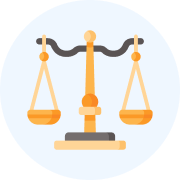CLAT  >  How to solve questions on Profit and Loss - 2

# How to solve questions on Profit and Loss - 2 Video Lecture - Quantitative Techniques for CLAT

## Quantitative Techniques for CLAT

46 videos|69 docs|95 tests

## FAQs on How to solve questions on Profit and Loss - 2 Video Lecture - Quantitative Techniques for CLAT

 1. How do I calculate the selling price if I know the cost price and the profit percentage?Ans. To calculate the selling price when you know the cost price and profit percentage, you can use the formula: Selling Price = Cost Price + (Profit Percentage/100) * Cost Price.
 2. What is the formula to calculate profit percentage?Ans. The formula to calculate profit percentage is: Profit Percentage = (Profit/Cost Price) * 100.
 3. How can I find the cost price if I know the selling price and the profit percentage?Ans. To find the cost price when you know the selling price and profit percentage, you can use the formula: Cost Price = Selling Price / (1 + (Profit Percentage/100)).
 4. How do I calculate the profit when I know the cost price and the selling price?Ans. To calculate the profit when you know the cost price and selling price, you can use the formula: Profit = Selling Price - Cost Price.
 5. Can you explain the concept of loss in profit and loss calculations?Ans. Loss occurs in profit and loss calculations when the selling price is less than the cost price. The loss is calculated as the difference between the cost price and the selling price. It is important to note that loss is represented by a negative value in profit and loss calculations.

## Quantitative Techniques for CLAT

46 videos|69 docs|95 testsExplore Courses for CLAT examSignup to see your scores go up within 7 days! Learn & Practice with 1000+ FREE Notes, Videos & Tests.
10M+ students study on EduRev
Track your progress, build streaks, highlight & save important lessons and more!
Related Searches

,

,

,

,

,

,

,

,

,

,

,

,

,

,

,

,

,

,

,

,

,

;